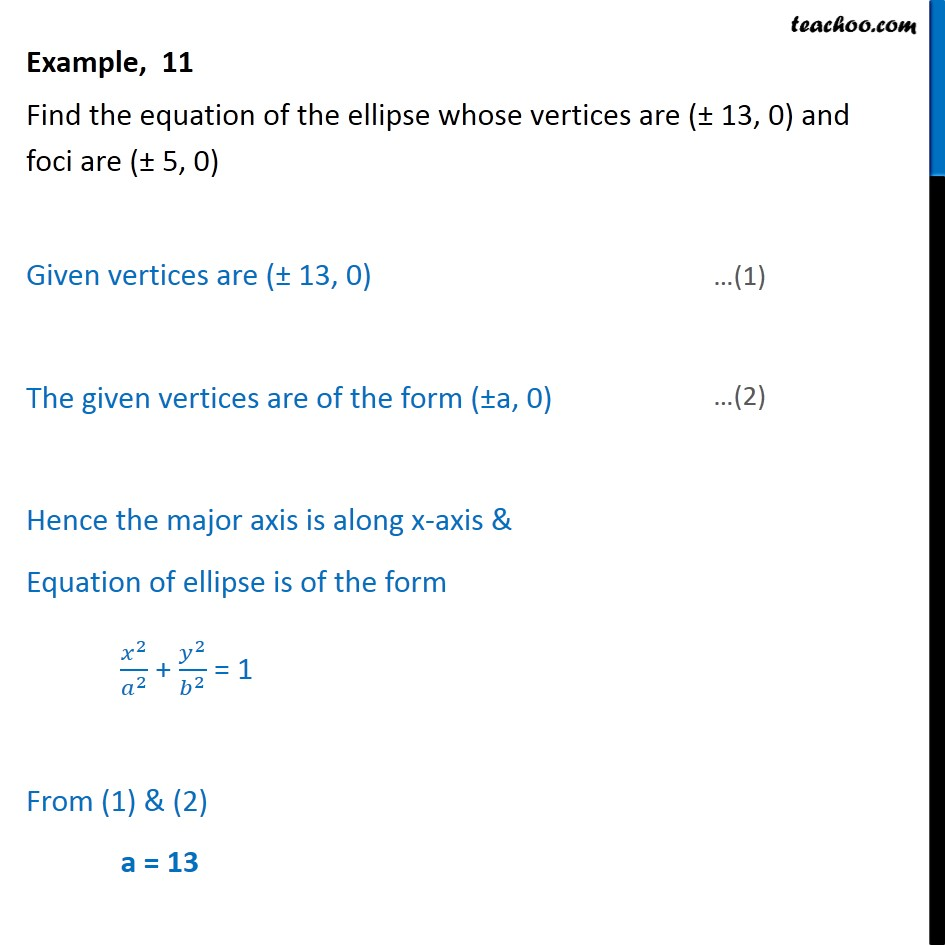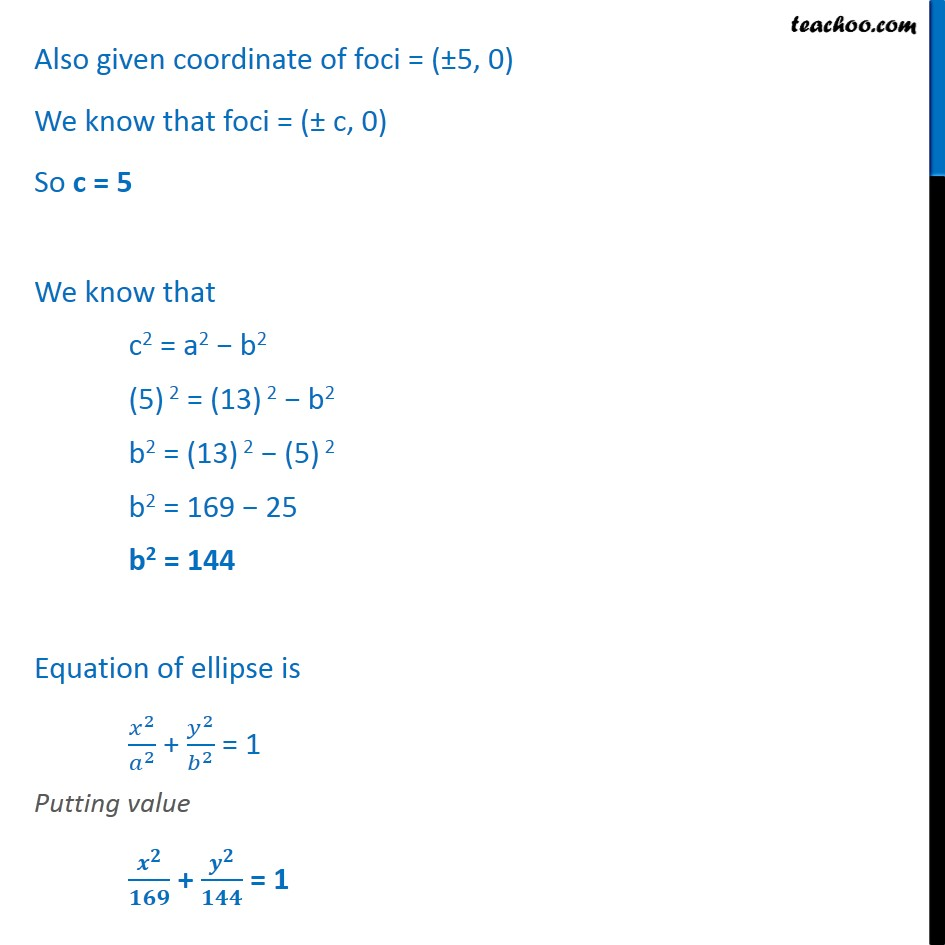Ellipse - Defination

Chapter 10 Class 11 Conic Sections
Concept wiseLearn in your speed, with individual attention - Teachoo Maths 1-on-1 Class

### Transcript

Example, 11 Find the equation of the ellipse whose vertices are (± 13, 0) and foci are (± 5, 0) Given vertices are (± 13, 0) The given vertices are of the form (±a, 0) Hence the major axis is along x-axis & Equation of ellipse is of the form ﷐﷐𝑥﷮2﷯﷮﷐𝑎﷮2﷯﷯ + ﷐﷐𝑦﷮2﷯﷮﷐𝑏﷮2﷯﷯ = 1 From (1) & (2) a = 13 Also given coordinate of foci = (±5, 0) We know that foci = (± c, 0) So c = 5 We know that c2 = a2 − b2 (5) 2 = (13) 2 − b2 b2 = (13) 2 − (5) 2 b2 = 169 − 25 b2 = 144 Equation of ellipse is ﷐﷐𝑥﷮2﷯﷮﷐𝑎﷮2﷯﷯ + ﷐﷐𝑦﷮2﷯﷮﷐𝑏﷮2﷯﷯ = 1 Putting value ﷐﷐𝒙﷮𝟐﷯﷮𝟏𝟔𝟗﷯ + ﷐﷐𝒚﷮𝟐﷯﷮𝟏𝟒𝟒﷯ = 1